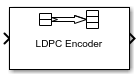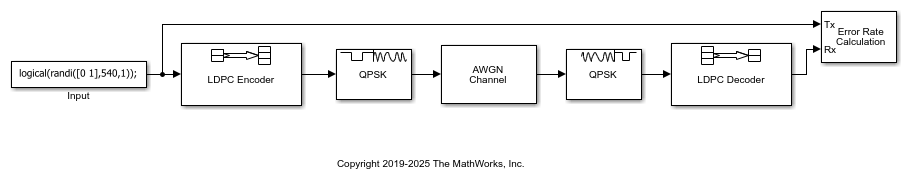# LDPC Encoder

Encode binary low-density parity-check (LDPC) code

•Libraries:
Communications Toolbox / Error Detection and Correction / Block

## Description

The LDPC Encoder block applies LDPC coding to a binary input message. LDPC codes are linear error control codes with sparse parity-check matrices and long block lengths that can attain performance near the Shannon limit.

The input and output are discrete-time signals. The ratio of the output sample time to the input sample time is K/N, where:

• N is the length of the received signal and must be in the range (0, 231).

• K is the length of the uncoded message and must be less than N.

## Examples

expand all

Transmit an LDPC-encoded, QPSK-modulated bit stream through an AWGN channel. Demodulate and decode the received signal. Compute the error statistics.

The `snr` variable is initialized using the `InitFcn` callback in Model Properties>Callbacks. The `SNR dB` parameter in the AWGN Channel block and the `Variance` parameter in the QPSK Demodulator Baseband block initialize settings using the `snr` variable.The simulation is configured to process one input frame of data. The Error Rate Calculation block compares the transmitted binary data stream with the information data bits output by the LDPC Decoder block.

```For SNR = 1 dB, the error rate is 0. ```

## Ports

### Input

expand all

Input message, specified as a K-by-1 column vector containing binary-valued elements. K is the length of the uncoded message.

Data Types: `double` | `Boolean`

### Output

expand all

LDPC codeword, returned as an N-by-1 column vector. N is the number of bits in the LDPC codeword. The output signal inherits its data type from the input signal. The LDPC codeword output is a solution to the parity-check equation. The input message comprises the first K bits of the LDPC codeword output, and the parity check comprises the remaining (NK) bits.

Data Types: `double` | `Boolean`

## Parameters

expand all

To edit block parameters interactively, use the Property Inspector. From the Simulink® Toolstrip, on the Simulation tab, in the Prepare gallery, select .

Parity-check matrix, specified as a sparse (NK)-by-N binary-valued matrix. N is the length of the output LDPC codeword and must be in the range (0, 231). K is the length of the uncoded message and must be less than N. The last (NK) columns in the parity-check matrix must be an invertible matrix in the Galois field of order 2, `gf`(2).

You can also specify the parity-check matrix as a two-column nonsparse index matrix, `I`, that defines the row and column indices of the `1`s in the parity-check matrix such that `sparse(I(:,1),I(:,2),1)`.

This parameter accepts numeric data types. When you set this parameter to a sparse binary matrix, this parameter also accepts the `Boolean` data type.

The default value uses the `dvbs2ldpc` function to configure a sparse parity-check matrix for half-rate LDPC coding, as specified in the DVB-S.2 standard.

Note

• When the last (NK) columns of the parity-check matrix form a triangular matrix, forward or backward substitution is performed to solve the parity-check equation.

• When the last (NK) columns of the parity-check matrix do not form a triangular matrix, a matrix inversion is performed to solve the parity-check equation. If a large matrix needs to be inverted, initializations or updates take more time.

Example: `dvbs2ldpc(R,'indices')` configures the index matrix for the DVB-S.2 standard, where `R` is the code rate, and `'indices'` specifies the output format of `dvbs2ldpc` as a two-column double-precision matrix that defines the row and column indices of the `1`s in the parity-check matrix.

## Block Characteristics

 Data Types `Boolean` | `double` | `integer` | `single` Multidimensional Signals `no` Variable-Size Signals `no`

## Version History

Introduced in R2007a